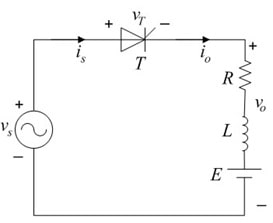# ELCT 704 Power Electronics Assignment 1

```Faculty of Information Engineering & Technology
Electrical & Electronics Department
Power Electronics (ELCT 704)
German University in Cairo
```A 220V, 50Hz voltage source is used to supply the armature circuit of an R-L-E load through a single phase half wave controlled rectifier. If the value of the resistance is 1Ω, the inductance is 0.015H and the battery voltage is 20V.

• Find an expression for the instantaneous output current in terms of the firing angle α and the extinction angle β. (No calculations are required)
• Find the firing angle at which the average output voltage becomes maximum.
• For the firing angle of Part (2), find the average output voltage and average current.
• For a firing angle of 90o, sketch the following:
1. The supply voltage and the battery voltage on the same graph
2. The output voltage
3. The output current
4. The voltage across the thyristor